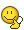BrainDen.com - Brain Teasers
• 0## Question(a-x)*(b-x)*(c-x)............(z-x)

No matter what any of the letters represent, they will always total the same answer. Why?

This might have already been posted. If yes, lock it.

0, because it will soon to be (x-x) and will multiply the whole equation by zero.

## Recommended Posts

• 0My first thought was, if you start the post with a spoiler, no one has a chance to be the first to guess it. Secondly, and I just joined so forgive me if I am out of line here, but that isn't technically an equation because there is no equal sign. What is it properly called?

##### Share on other sites
• 0Easy. 0

##### Share on other sites
• 00 was my initial thought but then i realised that it wasn't a*x it's A - x

##### Share on other sites
• 0It's not necessarily 0, let's say a=1, b=2, c=3...z=26 and x=50, in which case the answer is not 0...

##### Share on other sites
• 0THE ANS IS 0.

BCOZ THIS SERIE WILL ALSO INCLUDE (X-X).....WHICH LL MAKE THE WHOLE PRODUCT 0.

(a-x)*(b-x)*(c-x)............(z-x)

No matter what any of the letters represent, they will always total the same answer. Why?

This might have already been posted. If yes, lock it.

0, because it will soon to be (x-x) and will multiply the whole equation by zero.

##### Share on other sites
• 0It's not necessarily 0, let's say a=1, b=2, c=3...z=26 and x=50, in which case the answer is not 0...

x-x=50-50=0.##### Share on other sites
• 0v-w-x-y-z:duh: I forgot x was part of the alphabet letters...Edited by Anza Power

##### Share on other sites
• 0in those terms u have a term (x-x) which is zero and so product always shows up a zero

##### Share on other sites
• 0.....(x-x) =0

Hence the answer is 0

##### Share on other sites
• 0If x is the same as any of the variables a-z, then that expression in parentheses will =0 and 0 times all the rest will equal 0.

##### Share on other sites
• 0My first thought was, if you start the post with a spoiler, no one has a chance to be the first to guess it. Secondly, and I just joined so forgive me if I am out of line here, but that isn't technically an equation because there is no equal sign. What is it properly called?

'Better wise than smart', the term is called an expression.

It does matter what the letters represent.

For Lawlpie's solution to be correct, the x should be termed a constant, parameter, or variable. And, perhaps the expression be termed an algebraic expression.

With the loose phrasing of the problem, we could let x represent a function that makes the given solution invalid. Such as, let x be equal to F(x) where F(x) = -n, such that n is equal to the positional occurance of the variable (letter) x in the expression. Let the value of each of the other variables equal 0.

As we begin evaluating left-to-right, (0-(-1))*(0-(-2))*(0-(-3))*..., we see we have a positive progression that is similar to that of a factorial; and, when we reach the 24rd bracketed term, (x - x), we find it does not evaluate to 0, but to 1 = ((-24) - (-25)). The 25th and 26th bracketed terms evaluate to 26 and 27 respectively, thus, in this example, the expression evaluates to 27!/(24*25), which equates to 18148115750697253601280000, and not to 0.

Edited by Dej Mar

## Join the conversation

You can post now and register later. If you have an account, sign in now to post with your account.×   Pasted as rich text.   Paste as plain text instead

Only 75 emoji are allowed.

×   Your previous content has been restored.   Clear editor

×   You cannot paste images directly. Upload or insert images from URL.

×